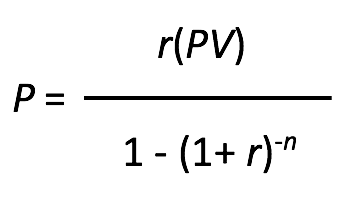The potential annual savings amount shown in results are calculated using the below formula and assumes 12 monthly repayments using the lowest Canstar 5-Star Rated home loan rate based on the information you have provided. The calculation formulae for home loan repayments are as follows:

Principal & interest:where:

P = the repayment amount;

PV = the original/initial loan amount;

r = the advertised interest rate (p.a.), divided by the number of repayments per year (repayment frequency); and

n = the total loan term in years, multiplied by the frequency of repayments per year.

Interest-only:where:

P = the repayment amount;

PV = the original/initial loan amount; and

r = the advertised interest rate (p.a.), divided by the number of repayments per year (repayment frequency).